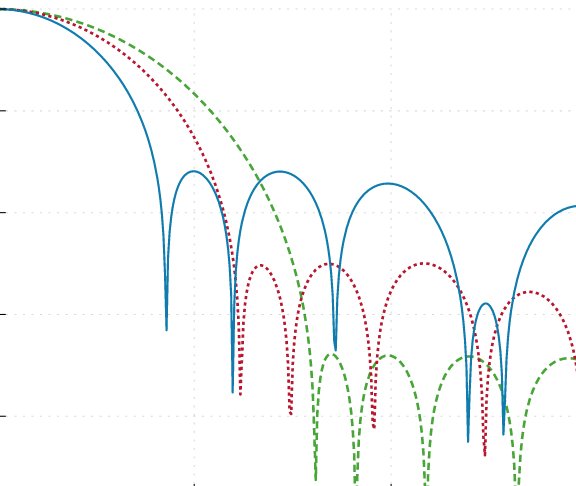Geraint Luff
Signalsmith Audio Ltd.
original

We combine box-filters to create a family of cheap FIR smoothing filters, and provide some optimal sizes.## Rectangular moving averages

We previously looked at box-filters (a.k.a. rectangular moving average), which can be used to smooth a signal:Note that the average settles exactly in finite time (100 samples) when the input signal goes to 0.

Smoothing filters generally remove high-frequency content (so often take the form of a lowpass). This rectangular moving-average filter has some potentially useful properties:

If we look at the frequency-response, it's less impressive. The rectangular filter lets through quite a lot of the high frequencies:We have normalised the time and frequencies - essentially showing the continuous-time equivalent. We discuss some of the practical details for sampled audio later.
Brief comparison with one-pole IIR

If you're wondering how this FIR moving-average compares to a first-order IIR filter, here you go:

To achieve a similar amount of smoothing (high-end reduction), the one-pole filter's impulse response has higher peaks and takes longer to settle. There are situations where both of these are awkward. On the other hand, the frequency response of one-pole IIRs is monotonic, which is sometimes useful.

Higher-order IIR filters with monotonic step-response (non-negative impulse) are a really cool area, but that's a subject for another article.

We can see the problem in the time-domain if we try to smooth out a sawtooth wave - the result still has hard corners:

Can we get a smoother result, corresponding to a better lowpass?

Using a box-filter smoothed our signal a bit, but not completely - so let's just do the same thing again!

If we cascade multiple shorter box-filters in a row, our impulse-response is the same total length as before, but the high frequencies fall off faster:

In terms of high-frequency suppression, this is looking good - but we can do better..

Our box-filters don't have to be equal length, and we can improve both the main-lobe width and side-lobe peaks by using uneven sizes:

The main lobe (or "passband" if we're considering this as a lowpass) gets wider the more stages we have, but the side-lobes (rejection band) get lower.

### Finding the lengths

I wish I had a neat solution to finding these ratios. Instead, I found them through numeric search, looking at the frequency-domain. To start, I placed all the lengths with 2x of each other, like this:

From this starting point, it was a straightforward hill-climbing optimisation to reduce the maximum peak within that range. Here are some of my results:

There are results up to depth 20 here.

## Real-world use

Let's take a look at how some of these cascaded filters perform on the sawtooth input:The filter is linear-phase, but has some latency/delay - which is why the filtered signal's zero-crossings are 50 samples later than the input's.

### Impact of wider passband

As you can see in the graph above, the depth-4 cascade produces results that oscillate more than the depth-2, even though the oscillations themselves are smoother.

This is the downside of our filter having a wider main-lobe (as seen in the analysis above). For the depth-4 cascade, the sawtooth's fundamental frequency lands within the passband, and is therefore partially preserved in the output.

To get the same passband width with a deeper stack, you would need to use a longer filter.

### Rounding the sizes

We showed the results above for continuous time - but in practice, each box-filter in our cascade will be to be of integer length. This means that deeper cascades with shorter lengths will be further from the ideal length ratios, leading to higher peaks in the stop-band:Compare these results (particularly for depths 3+) against ideal version (which was actually calculated with length 1024).

So, for the ratios above, the goal is to have the orders approximately in those ratios, not the lengths.

## Conclusion

I think these FIR smoothing filters are pretty cool, and it's satisfying to have some optimal sizes instead of guessing. The fact that their CPU cost is independent of their length is also pretty convenient.

I've used these a lot already for smoothing gain envelopes (where overshoot would be a problem, but high-frequency content would cause intermodulation) - I hope you find them useful as well!